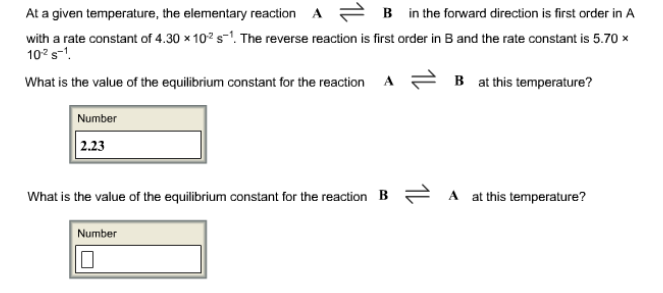# Problem: At a given temperature, the elementary reaction A ⇌ B in the forward direction is first order in A with a rate constant of 4.30 x 102 s-1. The reverse reaction is first order in B and the rate constant is 5.70 x 102 s-1.What is the value of the equilibrium constant for the reaction A  ⇌ B at this temperature? What is the value of the equilibrium constant for the reaction B ⇌ A at this temperature?

###### FREE Expert Solution
81% (499 ratings)###### Problem Details

At a given temperature, the elementary reaction A ⇌ B in the forward direction is first order in A with a rate constant of 4.30 x 102 s-1. The reverse reaction is first order in B and the rate constant is 5.70 x 102 s-1.

What is the value of the equilibrium constant for the reaction A  ⇌ B at this temperature?

What is the value of the equilibrium constant for the reaction B ⇌ A at this temperature?